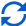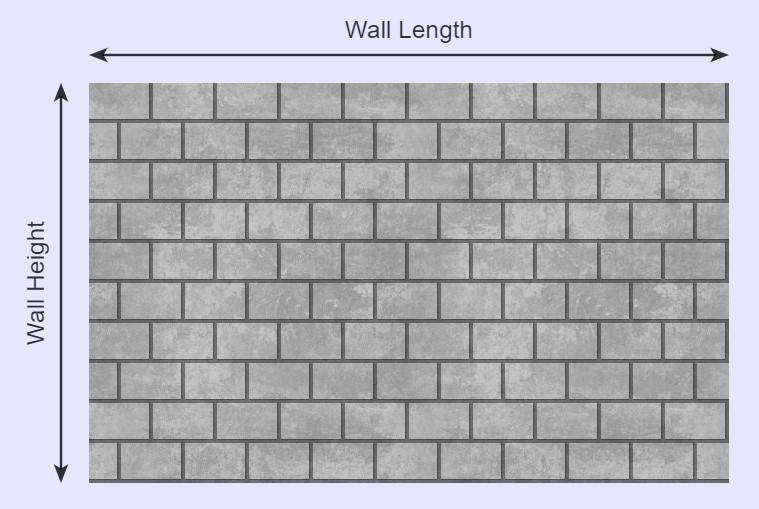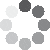Enter parameters
Enter parameters
Wall Length:
ft
• inches (in)
• feet (ft)
• yards (yd)
• centimeters (cm)
• meters (m)
Wall Height:
ft
• inches (in)
• feet (ft)
• yards (yd)
• centimeters (cm)
• meters (m)
Brick Length:
in
• inches (in)
• feet (ft)
• yards (yd)
• centimeters (cm)
• meters (m)
Brick Height:
in
• inches (in)
• feet (ft)
• yards (yd)
• centimeters (cm)
• meters (m)
Mortar joint:
in
• inches (in)
• feet (ft)
• yards (yd)
• centimeters (cm)
• meters (m)
Price Per Brick:
Waste (%):Result
Wall Area:
0
ft²
• square feet (ft²)
• square inches (in²)
• square yards (yd²)
• square miles (mi²)
• acres (ac)
• square centimeters (cm²)
• square meters ()
Bricks:
0
Cost:
$(1 votes, average: 5.00 out of 5)Loading... Similar Calculators: Real-time graphics. Make the calculations and see the changes. Embed Similar Calculators:When starting any home improvement or outdoor landscaping project, it is vital that you have an idea of how much material the job will need. Being prepared and understanding the requirements of the project is vital to its success. Imagine laying the foundation for a building and not knowing how many concrete cinder blocks you would need. That’s why we made our concrete block calculator. 😎 In this article we will show you how you can use it to make an efficient start on your next landscaping project. We will also explain that Mathematics the calculator uses to work out the total wall area, number of blocks and total estimated cost for the masonry project. Contents: ## Concrete block calculator formulaThe calculator first works out the area of the wall based on the measurements you provide: $$Wall\,Area = Wall\,Length \times Wall\,Height$$ and the area of each concrete block (otherwise known as a cinder block or cmu): $$Block\,Area = Block\,Length \times Block\,Height$$ ### Calculating the Total Number of Concrete Blocks Required In order to calculate the quantity of concrete blocks your wall will require, you need to know the distance between each concrete block. This is known as the mortar joint, and is usually given in inches. As a general rule, we use mortar between bricks, stones, blocks etc. and cement for structural projects such as setting posts or foundations for a building. You will also need to make allowances for broken blocks, known as wastage. In general, block wastage is commonly between 15% for a hard block and 5% for softer ones. To calculate the total number of concrete blocks needed: $$Number\,of\,Blocks = {Wall\,Area \over (Block\,Length + Mortar\,Joint) \times (Block\,Height + Mortar\,Joint)} + Waste\,(\%)$$ ### Calculating the Total Cost of the Blocks The calculator can estimate the total price of the concrete blocks if you know the price per block using the formula: $$Cost = Number\,of\,Blocks \times Price\,Per\,Block$$ In addition, if you don’t know the price per concrete block, it can easily be calculated: $$Price\,Per\,Block = {Total\,Cost\,of\,Blocks \over Number\,of\,Blocks}$$ Something not clear? 😕 See below for a fully worked example where we demonstrate how you can use the concrete block calculator to work out how many concrete blocks are needed and the total cost of the building project. Otherwise, get your next home improvement project off to an efficient start with our free online block calculator! ## Example calculations Let’s say that I wanted to build a wall using concrete blocks measuring 16 inches in length and 8 inches high with a mortar gap of half an inch. We want the wall to be 15 feet high and 30 feet in length. I want to know how many concrete blocks will be required, and an estimate for the cost of the masonry project. Entering these dimensions into the concrete block calculator gives a wall area in square feet of: $$Wall\,Area = Wall\,Length \times Wall\,Height = 15\,ft \times 30\,ft = 450\,ft^2$$ and area of a concrete block in square inches of: $$Block\,Area = Block\,Length \times Block\,Height = 16\,in \times 8\,in = 128\,in^2$$ Let’s assume we have a block wastage of 12%. To calculate the total number of concrete blocks needed, the calculator does the following calculations: $$Number\,of\,Blocks = {Wall\,Area \over (Block\,Length + Mortar\,Joint) \times (Block\,Height + Mortar\,Joint)} + Waste\,(\%) = {450\,ft^2 \over (16\,in + 0.5\,in) \times (8\,in + 0.5\,in)} + 12\,(\%) = 517.47 \rightarrow 518\,blocks$$ Note the importance of rounding the number of blocks up. If you round to the nearest whole number, we would end up with an answer of 517 blocks, meaning we are slightly short! We want to calculate an estimate for the cost of this project. I don’t know the price per concrete cinder block, but I can purchase 75 blocks for a cost of$150.

Therefore:

$$Price\,Per\,Block = {Total\,Cost\,of\,Blocks \over Number\,of\,Blocks} = {150 \over 75} = 2\,per\,block$$

Therefore, the concrete estimator can work out an estimate for the total cost of the concrete cinder blocks:

$$Cost = Number\,of\,Blocks \times Price\,Per\,Block = 518 \times 2 = 1036$$

Finally, we imagine you are wondering:

## What if I want to work in other units?

Don’t worry. It’s very simple! 😉

Our calculator is able to convert between feet, inches, yards, centimeters and meters for you.

Simply select the desired units and the calculator will do the following conversions for you:

$$1\,foot = 12\,inches = 0.33\,yards = 30.48\,centimeters = 0.3048\,meters$$

$$1\,ft^2 = 144\,in^2 = 0.111\,yd^2 = 929\,cm^2= 0.0929\,m^2$$

Embed this calculator on your site!Add live graphics
Copied to clipboard! PreviewWhat's wrong?
Minimum 5 characters!